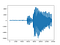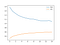# Speaker Recognition using TensorFlow

Recently, I work on some audio processing I found many example for Word Recognition; however, there was no recent example for Speaker Recognition. So, I propose you my implementation.

## Dataset

Code

`import csvimport ioimport osimport numpy as npimport randomimport globimport tensorflow as tffrom tensorflow.keras.models import Model, Sequential, load_modelfrom tensorflow.keras.layers import Input, Dense, BatchNormalization, Conv2D, MaxPooling2D, Dropout, Flatten, Reshape, AveragePooling2Dfrom tensorflow.keras.layers.experimental import preprocessingmodel_name = "model_speaker_reco"frame_length = 512 #1024 -> minimal freq ->86Hz for 44100Hz; 512 -> -> minimal freq ->62.5Hz for 16000Hzstep_length = 0.008image_width = 64 # 0.008*32=256ms …`

# Mining ETC (Ethereum Classic) and BTG (BitCoinGold) using GMiner

Winter is cold and I have an old GPU (AMD® Radeon (tm) r9 fury series); let’s mine to get warmer. First, I tried to use Xmrig to mine Monero, however, GPU mining is not efficient.

If you have a GPU, maximise your returns by mining a different coin (ETH, RVN, etc) on your GPU at the same time as mining Monero on your CPU. (https://minexmr.com/miningguide)

I tried many others mining softwere to use GPU and finnaly simplest one was GMiner.

## Installation

1. Download executable here: https://github.com/develsoftware/GMinerRelease/releases
2. Extract files
3. Update script for Coin you want to mine with your wallet address. For example…

# A journey to webcam realtime analysis

In this article, I will summarize my journey to webcam image and audio processing.

# Image processing

For image processing, we use cv2 module.

## Test webcam

`import cv2video = cv2.VideoCapture(1)#Change device number if neededfps = int(video.get(cv2.CAP_PROP_FPS))width = int(video.get(cv2.CAP_PROP_FRAME_WIDTH))height = int(video.get(cv2.CAP_PROP_FRAME_HEIGHT))print(fps, width, height)while True:    grabbed, frame = video.read()    print("====New frame====")    frame = cv2.resize(frame, (width//2, height//2))    cv2.imshow("Video", frame)    if cv2.waitKey(2) & 0xFF == ord("q"):        break`

## Detect face and create mean image

The mean image is created using the average pixel value of all detected faces during time. In next section, we will use this image as input of our machine prediction model.

`import cv2 import…`

# How to reduce the size of NumPy 1dim array

We often have to deal with very long lists of element, like audio data, and sometimes we want to reduce size to increase processing speed. There are many functions for image but not for 1dim array. I propose you my solution using only NumPy function.

# Here is my solution !

`def resize(inputArray, newLength):    block_size = len(inputArray)//newLength    outputArray = inputArray[0:block_size*newLength].reshape((block_size, newLength))    outputArray = np.mean(outputArray, axis=0)//np.max/np.min...    outputArray = outputArray.reshape((newLength))    return outputArray`

We reshape the array into a 2dims array constituted of block we will represent by one element using Mean, Max, Min or any function you want to use to reduce size of your array.

Note: this method only works to reduce size and cannot be used to increase the size of the array.

# Simple Voice Gender Recognition

When you heard about Gender Recognition you think about Image Processing; however, we can also identify gender using Voice.

# Let’s try!

First, check how we can do Gender Recognition using image.

Then, we can check some audio processing:

After that, we download audio dataset: https://commonvoice.mozilla.org/en/datasets

Dataset contains below labels including the gender (not for all files):

`client_id path sentence up_votes down_votes age gender accent locale segment`

# Let’s make something by ourselves

## Basic idea

We want to create a model which predict the gain to apply to noisy frequencies to close clear frequencies.

We use STFT to obtain spectrogram of the input, then we predict and apply the gain to it, and finally we use inverse STFT to reconstruct a waveform.

## Waveform to Spectrogram

`def audioToTensor(filepath:str): audio_binary = tf.io.read_file(filepath) audio, audioSR = tf.audio.decode_wav(audio_binary) audioSR = tf.get_static_value(audioSR) audio = tf.squeeze(audio, axis=-1) frame_step = int(audioSR * 0.008)#16000*0.008=128 spectrogram = tf.signal.stft(audio, frame_length=frame_length, frame_step=frame_step)#->31hz, si 512 -> 64hz spect_image = tf.math.imag(spectrogram) spect_real = tf.math.real(spectrogram) spect_sign = tf.sign(spect_real) spect_real = tf.abs(spect_real) return spect_real, spect_image, spect_sign, audioSR…`

# Comparison of Audio Representation in TensorFlow

This article compares the different Audio Representations implemented in TensorFlow and TensorFlowIO.

## Load the file

`audio = tfio.audio.AudioIOTensor(filepath)audioSR = int(audio.rate.numpy())audio = audio[:]audio = tf.squeeze(audio, axis=-1)audio = tf.cast(audio, tf.float32)`

# Oscillogram

`plt.figure("Oscillo: " + filepath)plt.plot(audio.numpy())plt.show()`Oscillogram of word “go”

Shape: (16000,)

# Spectrogram: tf.signal.stft()

`frame_step = int(audioSR * 0.008)spectrogram = tf.abs(tf.signal.stft(audio, frame_length=1024, frame_step=frame_step))plt.figure("Spect: " + filepath)plt.imshow(tf.math.log(spectrogram).numpy())plt.show()`

# Very simple Gender Recognition

In this article, I will try to provide you the simplest TensorFlow Gender Recognition implementation using TensorFlow.

## First, the data

We will use UTKFace Dataset.

## Then, the code

We use MobileNetV2 Model in order to keep the model small and usable on small devices.

`import tensorflow as tffrom tensorflow.keras.preprocessing.image import ImageDataGeneratorimport pandasimport globimage_size = (48, 48)batch_size = 32epochs = 15folders = ["UTKFace/"] countCat = {0:0, 1:0} class_weight = {0:1, 1:1} data, labels = [], [] for folder in folders: for file in glob.glob(folder+"*.jpg"): file = file.replace(folder, "") age, gender = file.split("_")[0:2] age, gender = int(age), int(gender)…`

# Very simple Emotion Recognition

In this article, I will try to provide the simplest TensorFlow Emotion Recognition implementation using TensorFlow.

## First, the data

We will use FER2013 Dataset.

## Then, the code

We use MobileNetV2 Model in order to keep the model light and usable on small devices.

`import tensorflow as tffrom tensorflow.keras.preprocessing.image import ImageDataGeneratorimage_size = (48, 48)batch_size = 32epoch = 15train_datagen = ImageDataGenerator(rescale=1./255, validation_split=0.2, horizontal_flip=True)test_datagen = ImageDataGenerator(rescale=1./255)train_generator = train_datagen.flow_from_directory(    "emotions/train",    target_size=image_size,    color_mode="grayscale",    shuffle=True,    batch_size=batch_size,    class_mode='categorical')validation_generator = test_datagen.flow_from_directory(    "emotions/test",    target_size=image_size,    shuffle=True,    color_mode="grayscale",    batch_size=batch_size,    class_mode='categorical')print(train_generator.class_indices)classifier = tf.keras.applications.mobilenet_v2.MobileNetV2(include_top=True, weights=None, input_tensor=None, input_shape=image_size + (1,), pooling=None, classes=7)classifier.compile(loss='categorical_crossentropy', metrics=['accuracy'])classifier.fit(train_generator, steps_per_epoch=train_generator.samples//batch_size, epochs=epochs, validation_data=validation_generator, validation_steps=validation_generator.samples//batch_size)classifier.save("emotion_model")`

## Folder structure

• /train_emotion.py
• /emotions/[test, train]/[angry, disgust, fear, happy, neutral, sad, surprise]/+.jpg

## Fitting result

I obtained an accuracy of 0.4873 after 15 epochs, however progression is not over.Fitting history

# A Journey to Speech Recognition Using TensorFlow

Nowadays, we can use high precision voice recognition in our smartphone or any smart devices. However, those systems are provided by big companies like Google, Amazon or Apple, and are not free.

Many people, including myself, thought that it was because of a lack of free data. However, nowdays, we can easily find free data on the Internet.

Voice datasets

Dataset sizes for some languages (2020/10/12)

• English → 50 GB
• German → 16GB
• French → 15GB
• Japanese → 265MB

Some other data here:

Tools

Maybe, it was because no tools are available…## Arnaud

Working in computer science.